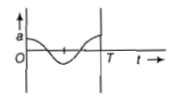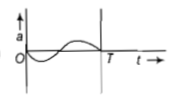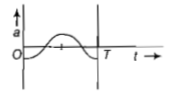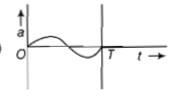• Subject:

...

• Topic:

...

The oscillation of a body on a smooth horizontal surface is represented by the equation, X = A cosωt where X=displacement at time t ω=frequency of oscillation
Which one of the following graphs shows correctly the variation a with t?
(a)(b)(c)(d)Here,a=acceleration at time t T=time period

NEET